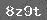# 六年级:“和差问题”的作用

问题：图上正方形ABCD是一条环形公路，已知汽车在每条边上行驶的速度分别为每小时：AB边上90千米、BC边上120千米、CD边上60千米、DA边上80千米。现从CD边上一点P同时反向出发两辆汽车，它们在AB边上中点相遇；如果从PC中点M同时反向出发两辆汽车，则它们在AB边上的N点相遇。已知AN之间的距离是10千米，求这个环形公路的总长度。本题的关键显然是求出AN与NB与正方形边长之间的关系，为此，先要求出DM与MC距离之间的关系，这可以通过DP与PC距离之间的关系求出。

如果设正方形的边长为单位1，那么两辆汽车在DC边上行驶的总时间为DP边上行驶所用时间多出来的部分，就是在AD边上行驶所用时间比在BC边上行驶所用时间多出来的部分，也就是：现在根据“和差问题”的计算公式：

大数=（和+差）÷2 小数=（和-差）÷2

就可以求出在DP边上所用时间为：在PC边上所用时间为：所以DP与PC的距离之比为MA上行驶所用时间为二者相差的时间为这个相差的时间就是AN与NB上行驶相差的时间，而AB边上行驶的总时AN与NB行驶所用时间分别为方形的边长为这个环形公路的总长度为

320×4=1280（千米）

至此，本题全部解决了。解题过程中两次利用了“和差问题”这一基本类型。学习数学特别强调对基本内容的理解和掌握，原因就是许多复杂问题的解决依赖于对基本内容掌握的程度。希望同学们一定要重视“基本”。下面再来解决一个问题。

问题 下面的方格中填上加号或减号，使得算式的结果等于23。

9□8□7□6□5□4□3□2□1=23

首先不难发现所有出现的数字总和为45。如果把所有前边为加号的数字归为一组，前边为减号的数字归为一组，这两组数字之和的差就是23。根据“和差问题”的公式，前边为加号的一组数字之和为

（45+23）÷2 =34

前边为减号的一组数字之和为

（45-23）÷2=11

现在只要挑选出哪些数字的和是11就可以知道哪些数字前边填减号，自然就可以知道哪些数字前边填加号了。数字和为11的情况有：

8+3=11 　　8+2+1=11

7+4=11　　 7+3+1=11

6+5=11 　　6+4+1=11

6+3+2=11 　5+3+2+1=11

5+4+2=11

相应的填法就有

9-8+7+6+5+4-3+2+1=23

9-8+7+6+5+4+3-2-1=23

9+8-7+6+5-4+3+2+1=23

9+8-7+6+5+4+3-2-1=23

9+8+7-6-5+4+3+2+1=23

9+8+7-6+5-4+3+2-1=23

9+8+7-6+5+4-3-2+1=23

9+8+7+6-5+4-3-2-1=23

9+8+7+6-5-4+3-2+1=23

本题的解法能够做到将所有的情况列举出来，而如果直接试验，可能会丢掉一些情况。

### 相关推荐

 留言与评论（共有 0 条评论）

 验证码：